### Number systems - Remainders, divisors - questionbank - Ascent Education - XAT 2020 , TANCET 2020 study material, correspondence course

 Home TANCET Classes GMAT Coaching Online GMAT Course CAT Classes TANCET Study Material
 TANCET '18 Classroom Program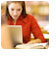Weekend and weekday classes for TANCET MBA @ Chennai. At Nungambakkam and Velachery.Other Courses TANCET Correspondence Course CAT Classes & Courses GMAT Classes Chennai Online GMAT Course GRE Classes Chennai CBSE Math Online Tuition SAT Classes Other Links Ascent TANCET Toppers Testimonials CAT, TANCET Questions Careers @ Ascent Contact Us +91 44 4500 8484 +91 96000 48484 ascent@ascenteducation.com Postal Address Facebook / Twitter / Blog / Videos
You are here: Home  »  XAT, TANCET Prep Questions »  Number Theory »   Remainders, divisors Question 5

# Remainders of division by a factor of 'd' : Number System

The question is a Number properties question. The question is about finding the remainder of the division of a number by a factor of a divisor 'd'; the remainder when a number is divided by the divisor 'd' is given.

#### Question

When a number is divided by 36, it leaves a remainder of 19. What will be the remainder when the number is divided by 12?
1. 10
2. 7
3. 19
4. 192
5. None of these
Correct Answer - 7. Choice (2)

Let the number be 'a'.

When 'a' is divided by 36, let the quotient be 'q' and we know the remainder is 19.

i.e.,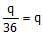and remainder is 19

or a = 36q + 19

when 'a' is divided by 12, we get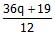or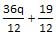.

36q is divisible by 12.

Therefore, remainder = 7

Level of difficulty : Moderate

## CAT, XAT, TANCET Practice Questions and Answers : Listed Topicwise

 Number Theory Permutation Combination Probability Inequalities Geometry Mensuration Trigonometry Coordinate Geometry Percentages Profit Loss Ratio Proportion Mixtures Alligation Speed Time Distance Pipes Cisterns Interest Races Average, Mean AP, GP, HP Set Theory Clocks Calendars Algebra Function English Grammar General Awareness Data sufficiency TANCET Papers XAT PapersAdd to del.icio.us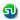Stumble Itdigg this